15 Math Puzzles and Number Tricks Kids Will Love
15 Math Puzzles and Number Tricks Kids Will Love

It can be hard to convince some kids that math is fun, but these magical math puzzles ought to do the trick! Enhance their logical thinking skills with engaging conundrums and clever numerical maneuvers. It may all seem like hocus pocus, but understanding the math behind it all makes it even more impressive!

## 1. “Pick a Number” Algebraic Math Puzzles

Let’s start with the classic “Pick a number, any number!” trick. Ask a student to follow these steps:

• Pick any number (We’ll use 73)
• Add 3 (73+3=76)
• Double the result (76×2=152)
• Subtract four (152-4=148)
• Divide that number in half (74)
• Subtract your original number (74-73=1)
• The answer is always 1!

Tricks like this are lots of fun for getting kids to practice mental math, but they also provide a terrific opportunity for kids to use algebraic thinking to come up with their own puzzles. Visit the link to learn a cool visual method to walk students through the steps.

Learn more: Elementary Math at EDC

## 2. Magic Squares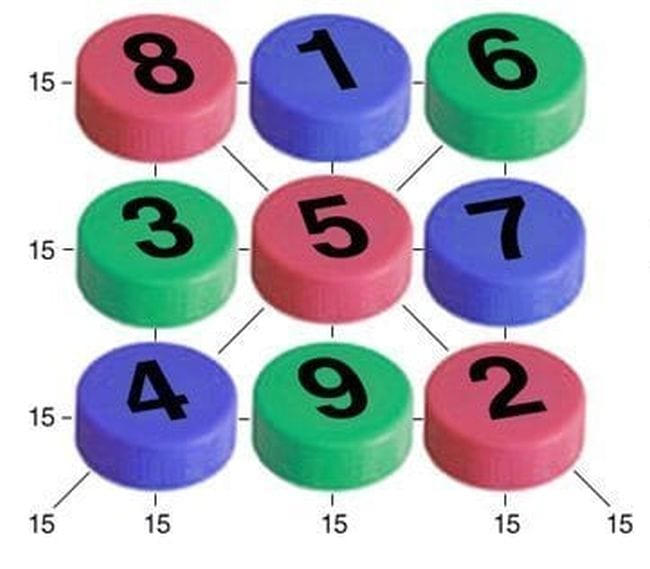Magic squares are the basis for the ever-popular Sudoku math puzzles, and they’re fantastic learning tools for kids. A magic square is made up of equal rows of numbers (3×3, 4×4, etc.). Each line of the square (horizontal, vertical, and diagonal) must add up to the same sum, and each box must contain a different number. For a 3×3 square, each line adds up to 15. For 4×4, each line equals 34.

Tip: To make it easier for kids to work out the solution to magic squares, try writing numerals on bottle caps. Now kids can slide them around until they get the right combination. Find out how these math puzzles work and get free printables at the link.

Learn more: Dad’s Worksheets

## 3. Magic Triangles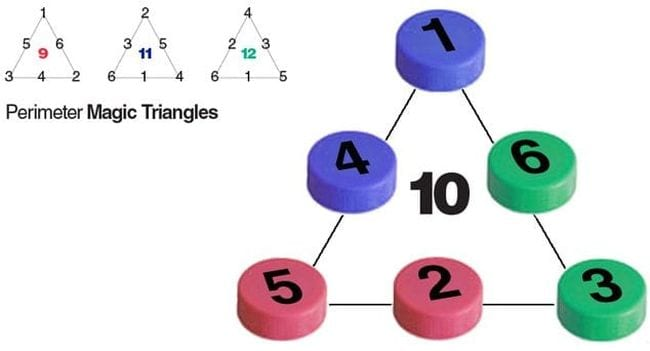Magic Triangles are just like magic squares, but each side of the perimeter adds up to the same number. This can be a low-key way to ease kids into magic squares, since there aren’t as many lines to contend with. Bottlecaps work perfectly for these math puzzles too!

Learn more: CueMath

## 4. Yohaku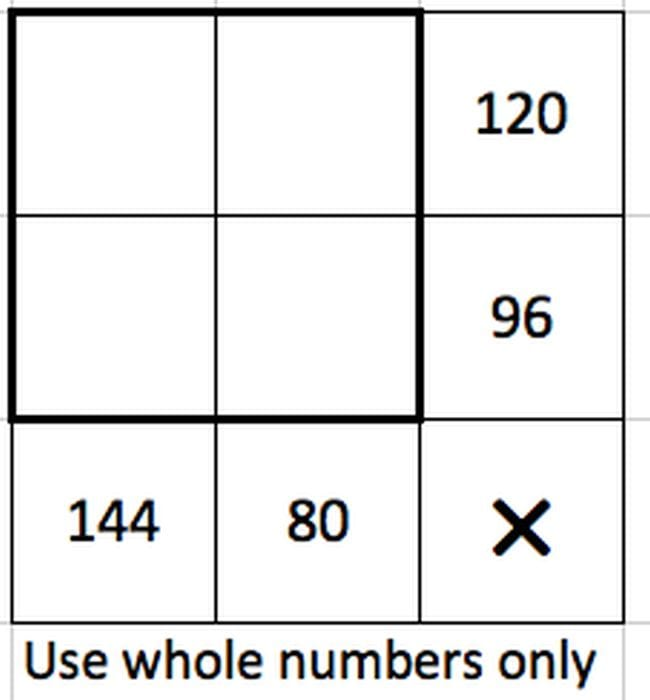Yohaku math puzzles are a new spin on magic squares. The challenge is to fill in the blank squares using the operation indicated in the bottom right corner. Each row and column must equal the numbers at the end. Find out how it works and get lots of free puzzles to try at the link.

Learn more: Yohaku

## 5. Calendar Magic 9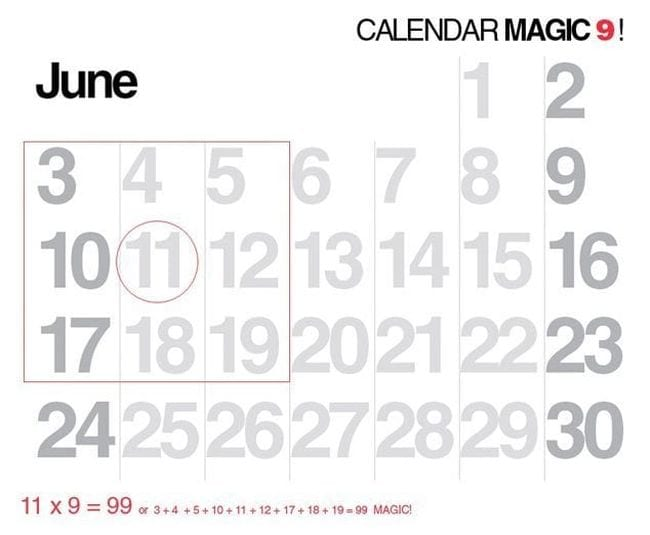Pull out a calendar and ask students to put a square around a 3 x 3 box, enclosing 9 numbers. Tell them you can find the sum of those 9 numbers faster than they can add it up on a calculator. All you have to do multiply the center number by 9—you’ll get the right answer every time!

Bonus Trick: Multiplying numbers by 9 is easy. Simply multiply the number by 10, and subtract the original number. For instance, say you want to multiply 9 x 17. Multiply 10 x 17 (170) and subtract 17 (153). Ta-dah!

Learn more: Calendar Math/Learn With Math Games

## 6. Number Pyramids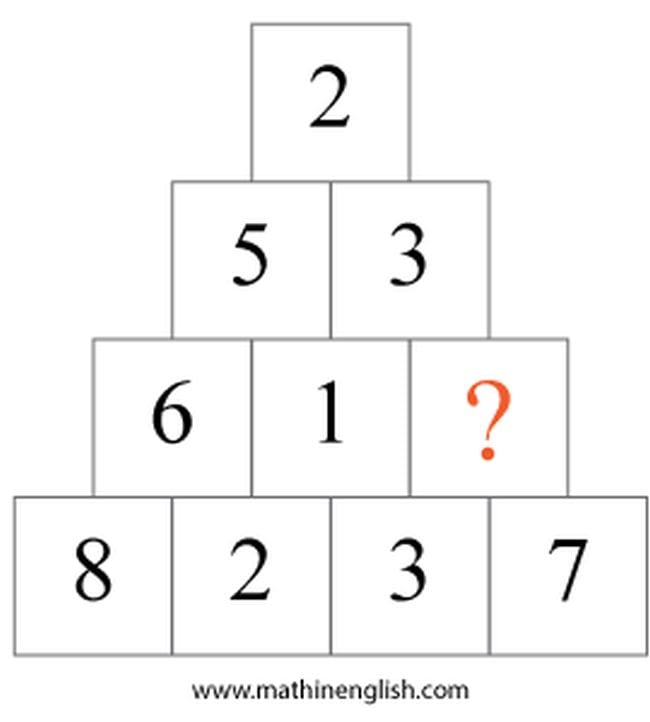In a number pyramid, numerals are arranged in patterns, and one or more squares are left empty to be filled in with the correct answer(s). In this one, each number is found by subtracting the smaller from the larger of the two numbers underneath. For example 8-2=6 and 5-3=2. The correct answer here is 7-3, which equals 4. Try this one on your students, then see if they can create their own math pyramids!

Learn more: Math in English

## 7. Math Crossword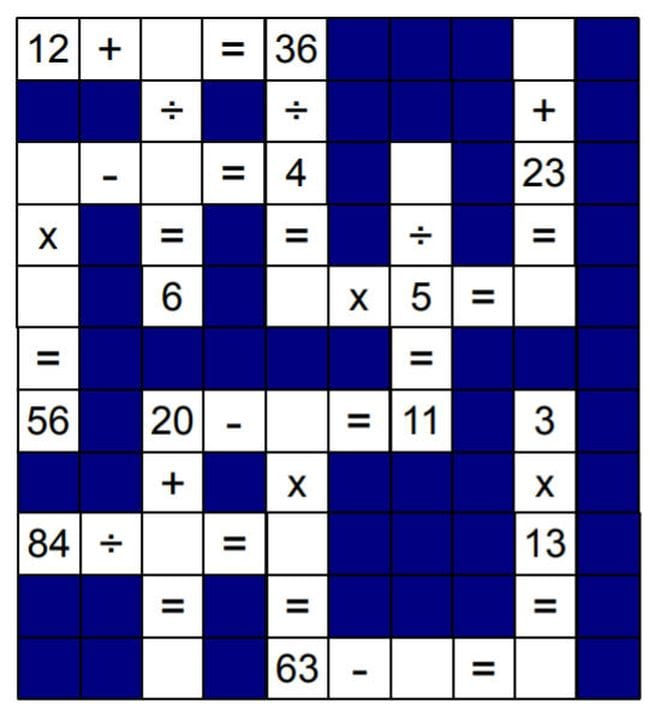Switch things up with a crossword made up of numbers and equations instead of letters! Have kids solve this one, then challenge them to make up one of their own.

Learn more: Math Crossword/Education.com

## 8. Magic Math Cards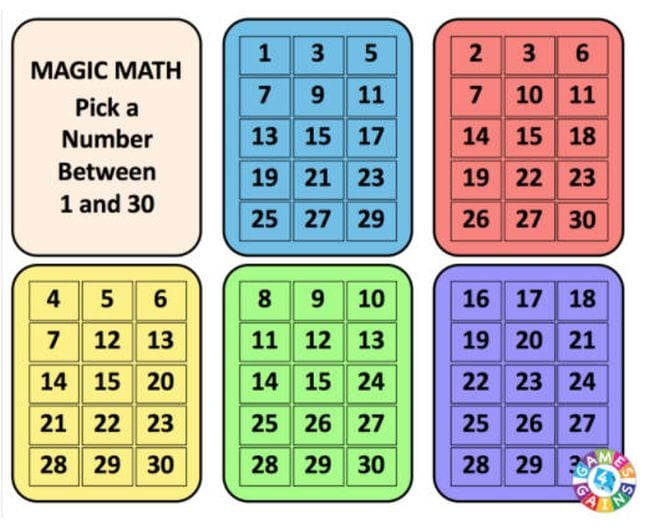Print the free cards at the link and use them for this clever “magic” trick. Put the cards in a pile and ask a student to pick any number between 1 and 30, without telling you what it is. As you show them each card one by one, you’ll ask them whether their number is on that card. If they say yes, note the number in the top left corner. Keep a running sum of those numbers, and announce your total at the end. That will be your student’s number!

Now ask kids if they can figure out how the trick works. You’ll find the answer at the link.

Learn more: Games 4 Gains

## 9. Toothpick Math Puzzles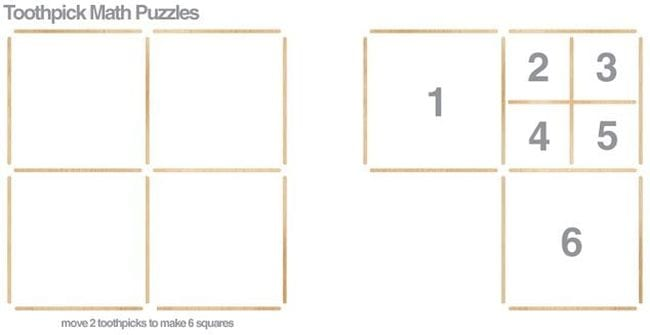Toothpick puzzles encourage logical thinking skills and geometry concepts too. Pass out a few boxes, then have kids arrange 12 toothpicks as shown to make 4 squares. Ask them to figure out how they can move only two toothpicks to make 6 squares. The answer is simple once you see it, but it requires kids to make a leap and recognize not all the squares need to be the same size.

Find 19 more toothpick puzzles at the link. For even more fun, ask kids to create their own toothpick math puzzles!

Learn more: Toothpick Geometry PDF/San Francisco State University

## 10. Deleting Sheep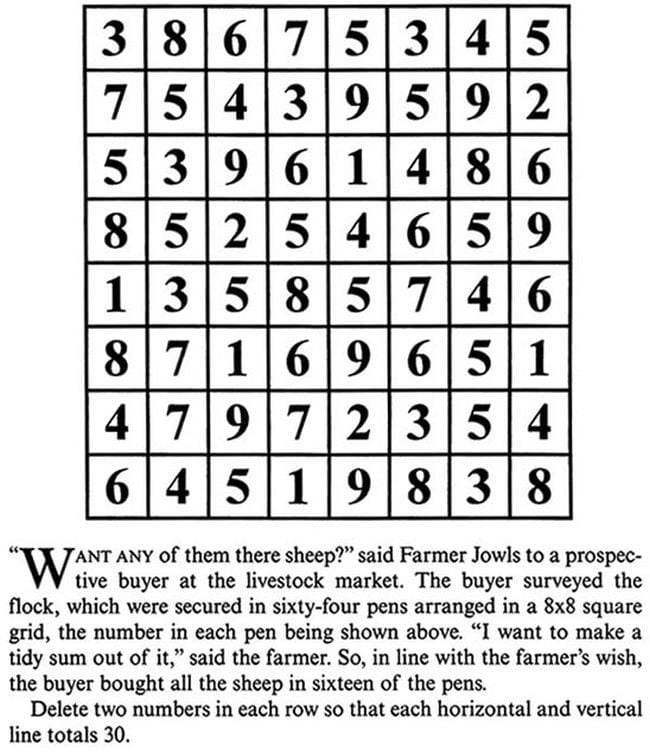Here’s a puzzle that will keep your students busy for quite some time. They’ll need to add up the numbers in each row and column and figure out by how much the total exceeds 30. Then, they need to eliminate 2 numbers in each row so the totals (horizontal and vertical) equal 30. The answer is at the link.

Learn more: Dover Publications (Answer 2)

## 11. Art in Numbers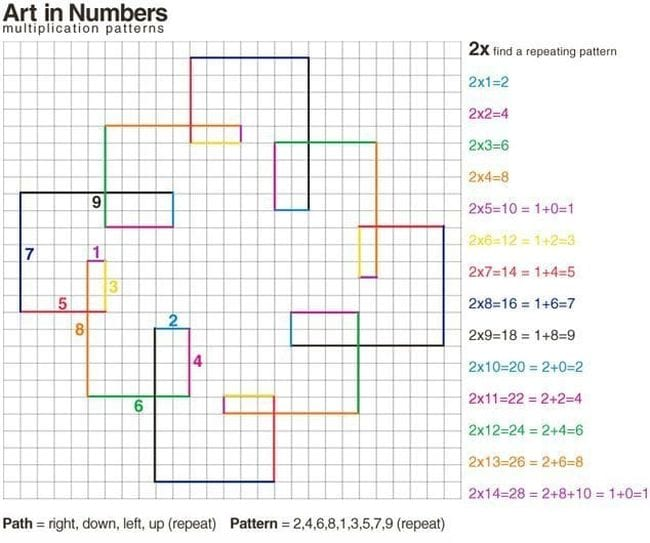Practice multiplication facts by creating graph paper designs called spirolaterals. When they see them visually, kids will learn to identify patterns in their multiplication tables.

Learn more: SharynIdeas

## 12. Two Circles Into a Square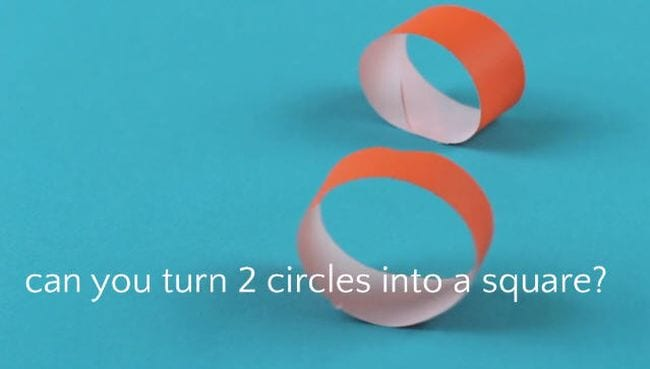Given two loops of paper chain and a pair of scissors, can kids figure out how to change them into a single square? The answer (video walk-through included) is at the link.

Learn more: What We Do All Day

## 13. Domino Math Puzzles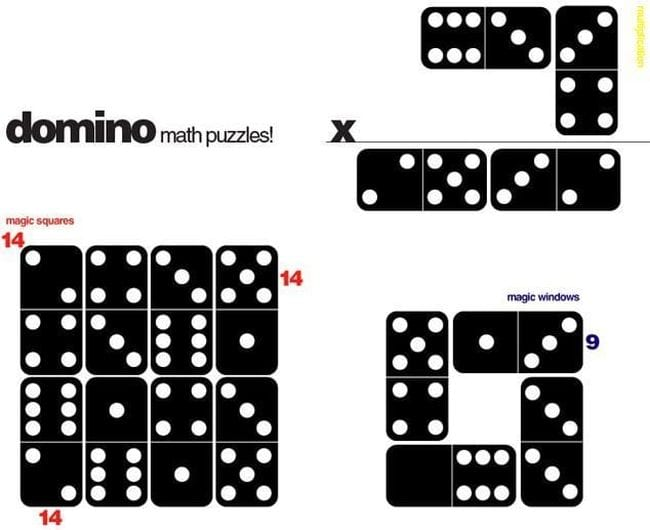The game of dominos is really one big math puzzle all on its own, but there are lots of other cool math tricks you can do with them! You can arrange them in magic squares and rectangles, lay out multiplication problems, set up magic windows, and more. Visit the link to learn how they work and find more ideas.

Learn more: NRICH

## 14. Subtraction Squares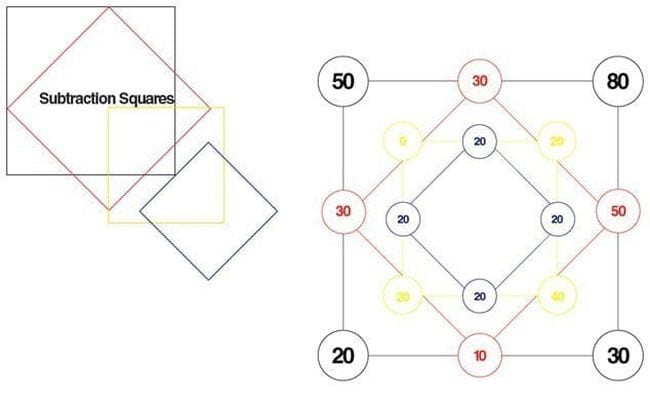Try this fascinating math trick to amaze your students! Grab the free printable puzzle blank at the link below. Then follow these steps.

1. Choose any four numbers and write them in the corner circles.
2. Start with the top horizontal line. Subtract the smaller corner from the larger one and write the difference in the middle circle. Repeat with the remaining sides.
3. Now repeat step 2 with the numbers in the next smaller square.
4. Continue until you reach the smallest square. In this one, all the corners will have the exact same number!

Learn more: Subtraction Squares/Learn With Math Games

## 15. The Answer Is…

Here are a couple of quick math puzzles to share at the end of class. Students can take them home to amaze friends and family!

### The Answer Is 2

• Think of a whole number 1 through 10 (We’ll use 6)
• Double it (6×2=12)
• Add 4 (12+4=16)
• Divide by 2 (16÷2=8)
• Subtract the original number (8-6=2)
• The answer is always 2!

### The Answer is 18

• Choose any number (We’ll use 31)
• Multiply the number by 100 (31×100=3100)
• Subtract the original number from the answer (3100-31=3069)
• Add those individual numerals together (3+0+6+9=18)
• The answer is always 18!

### Plus, get all the latest teaching tips and tricks by signing up for our newsletters!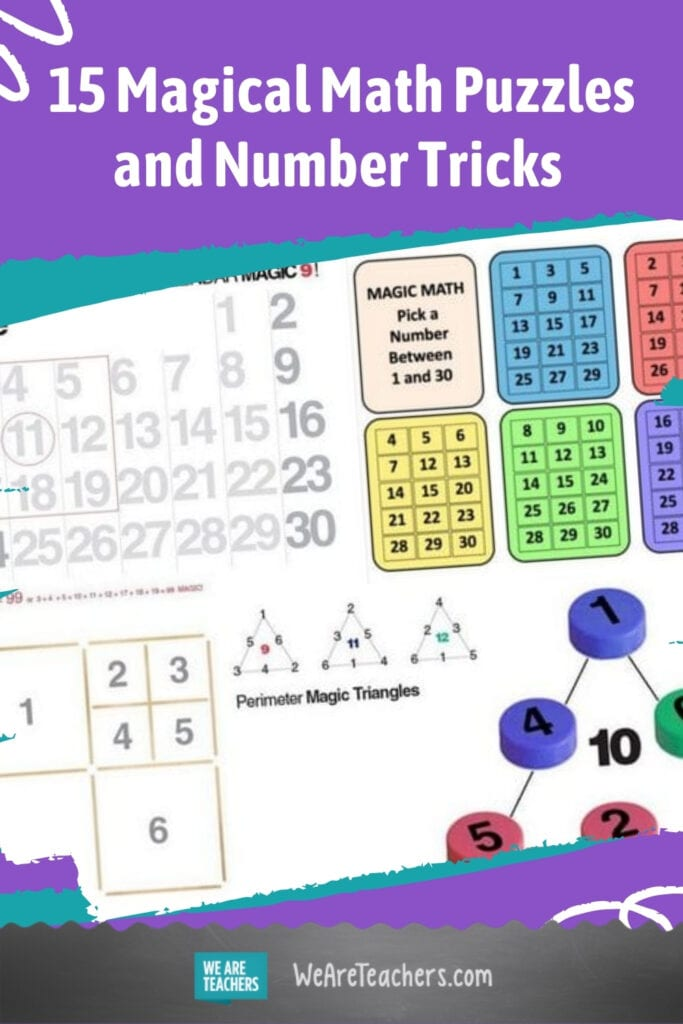Source link